# Quadratic equation + equation - math problems

#### Number of problems found: 290

• Column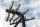Perpendicular pole high 8 m tall broke and its toe fell 2.7 m from the bottom of the pole. At what height above the ground pole broke?
• Circle and squareAn ABCD square with a side length of 100 mm is given. Calculate the radius of the circle that passes through the vertices B, C and the center of the side AD.
• RectangleThere is a rectangle with a length of 12 cm and a diagonal 8 cm longer than the width. Calculate the area of rectangle.
• Unknown number 4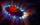I think number. When I divided its square root by its 1/9, I get a number 1. What number am I thinking?
• Three parallelsThe vertices of an equilateral triangle lie on 3 different parallel lines. The middle line is 5 m and 3 m distant from the end lines. Calculate the height of this triangle.
• Kohlrabies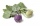The price of one kohlrabi increased by € 0.40. The number of kohlrabies that a customer can buy for € 4 has thus decreased by 5. Find out the new price of one kohlrabi in euros.
• Area and perimeter of rectangleThe content area of the rectangle is 3000 cm2, one dimension is 10 cm larger than the other. Determine the perimeter of the rectangle.
• Tangents to ellipseFind the magnitude of the angle at which the ellipse x2 + 5 y2 = 5 is visible from the point P[5, 1] .
• Cuboid wallsIf the areas of three adjacent faces of a cuboid are 8 cm², 18 cm² and 25 cm². Find the volume of the cuboid.
• Golden ratio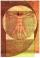Divide line of length 14 cm into two sections that the ratio of shorter to greater is same as ratio of greater section to whole length of the line.
• Sphere and coneWithin the sphere of radius G = 33 cm inscribe the cone with the largest volume. What is that volume, and what are the dimensions of the cone?
• Water reservoir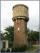The cuboid reservoir contains 1900 hectoliters of water and the water height is 2.5 m. Determine the dimensions of the bottom where one dimension is 3.2 m longer than the second one.
• Difference of legsIn a right triangle, the length of the hypotenuse is 65 m, and the difference of legs is 23 m. Calculate the perimeter of this triangle.
• Do you solve this?Determine area S of rectangle and length of its sides if its perimeter is 102 cm.
• Triangle ABC v2Area of the triangle is 12 cm square. Angle ACB = 30º , AC = (x + 2) cm, BC = x cm. Calculate the value of x.
• Pumps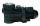The tank is filled with two pumps in 16 minutes. The first pump is filled in 30 minutes earlier than two one. How many minutes is filled with the first pump?A domed stadium is in the shape of spherical segment with a base radius of 150 m. The dome must contain a volume of 3500000 m³. Determine the height of the dome at its centre to the nearest tenth of a meter.
• AP RT triangleThe length of the sides of a right triangle form an arithmetic progression, longer leg is 24 cm long. What are the perimeter and area?
• Cuboid and eq2Calculate the volume of cuboid with square base and height 6 cm if the surface area is 48 cm2.
• Hypotenuse and heightIn a right triangle is length of the hypotenuse c = 56 cm and height hc = 4 cm. Determine the length of both trangle legs.

Do you have an interesting mathematical word problem that you can't solve it? Submit a math problem, and we can try to solve it.

We will send a solution to your e-mail address. Solved examples are also published here. Please enter the e-mail correctly and check whether you don't have a full mailbox.

Please do not submit problems from current active competitions such as Mathematical Olympiad, correspondence seminars etc...

Looking for help with calculating roots of a quadratic equation? Do you have a linear equation or system of equations and looking for its solution? Or do you have quadratic equation?